# Binary Hex Binary and Hex The number systems

• Slides: 22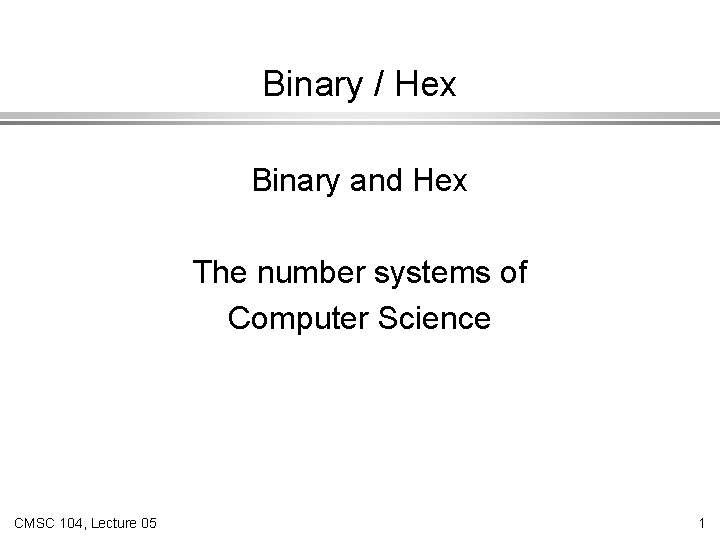Binary / Hex Binary and Hex The number systems of Computer Science CMSC 104, Lecture 05 1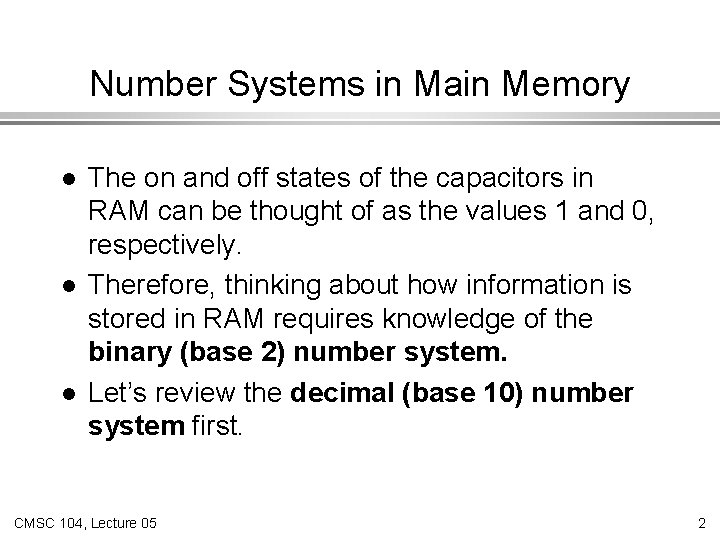Number Systems in Main Memory l l l The on and off states of the capacitors in RAM can be thought of as the values 1 and 0, respectively. Therefore, thinking about how information is stored in RAM requires knowledge of the binary (base 2) number system. Let’s review the decimal (base 10) number system first. CMSC 104, Lecture 05 2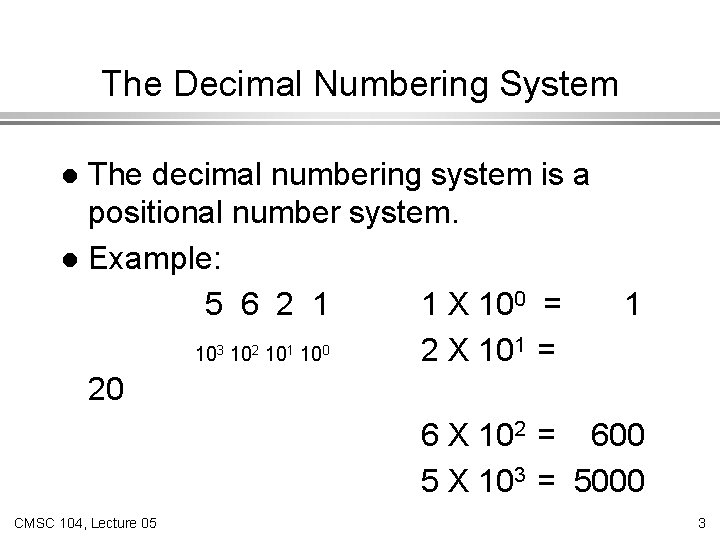The Decimal Numbering System The decimal numbering system is a positional number system. l Example: 5 6 2 1 1 X 100 = 1 103 102 101 100 2 X 101 = 20 6 X 102 = 600 5 X 103 = 5000 l CMSC 104, Lecture 05 3What is the base ? The decimal numbering system is also known as base 10. The values of the positions are calculated by taking 10 to some power. l Why is the base 10 for decimal numbers ? l Because we use 10 digits: the digits 0 through 9. CMSC 104, Lecture 05 4The Binary Number System The binary numbering system is also known as base 2. The values of the positions are calculated by taking 2 to some power. l Why is the base 2 for binary numbers ? l Because we use 2 digits: the digits 0 and 1. CMSC 104, Lecture 05 5The Binary Number System (con’t) l l l The Binary Number System is also a positional numbering system. Instead of using ten digits, 0 - 9, the binary system uses only two digits, 0 and 1. Example of a binary number and the values of the positions: 1 0 0 0 1 2 6 2 5 2 4 23 2 2 21 2 0 CMSC 104, Lecture 05 6Converting from Binary to Decimal 1 0 0 1 1 0 1 26 2 5 2 4 2 3 2 2 2 1 2 0 20 = 1 21 = 2 22 = 4 23 = 8 CMSC 104, Lecture 05 24 = 16 25 = 32 26 = 64 1 X 20 = 1 0 X 21 = 0 1 X 22 = 4 1 X 23 = 8 0 X 24 = 0 0 X 25 = 0 1 X 26 = 64 77 7Converting from Binary to Decimal (con’t) Practice conversions: Binary Decimal 11101 1010101 100111 CMSC 104, Lecture 05 8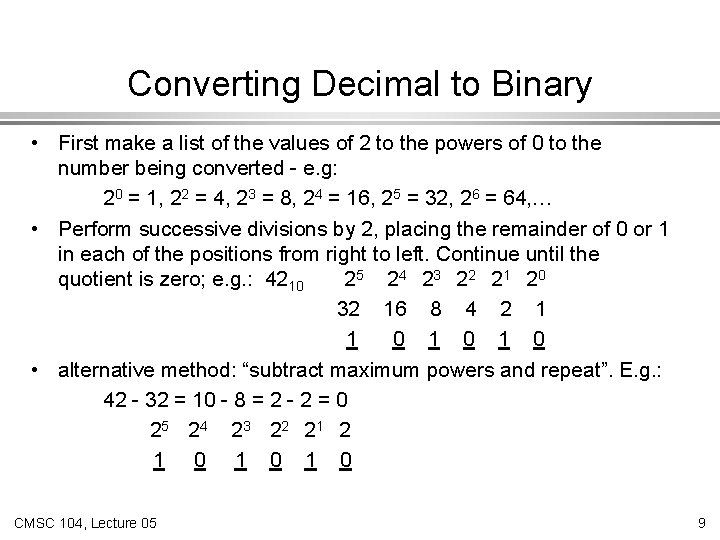Converting Decimal to Binary • First make a list of the values of 2 to the powers of 0 to the number being converted - e. g: 20 = 1, 22 = 4, 23 = 8, 24 = 16, 25 = 32, 26 = 64, … • Perform successive divisions by 2, placing the remainder of 0 or 1 in each of the positions from right to left. Continue until the quotient is zero; e. g. : 4210 2 5 24 23 22 21 20 32 16 8 4 2 1 1 0 1 0 • alternative method: “subtract maximum powers and repeat”. E. g. : 42 - 32 = 10 - 8 = 2 - 2 = 0 2 5 24 23 22 21 2 1 0 1 0 CMSC 104, Lecture 05 9Converting From Decimal to Binary (con’t) Practice conversions: Decimal Binary 59 82 175 CMSC 104, Lecture 05 10Counting in Binary l Binary 0 1 10 11 100 101 110 111 CMSC 104, Lecture 05 l Decimal equivalent 0 1 2 3 4 5 6 7 11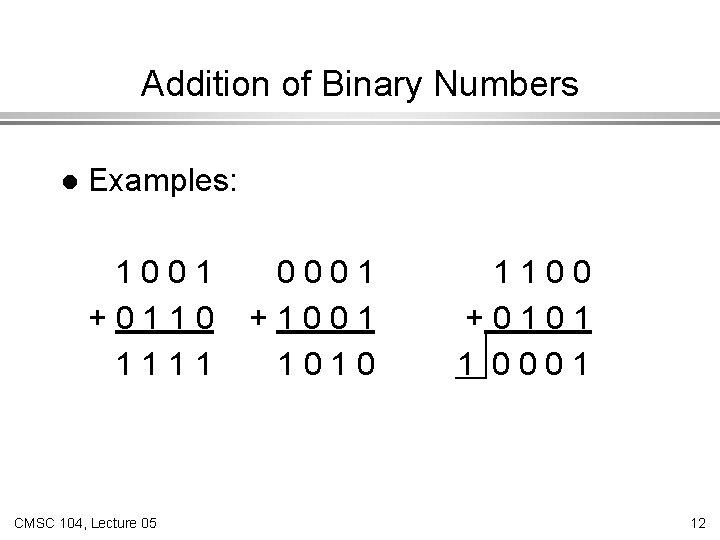Addition of Binary Numbers l Examples: 1001 +0110 1111 CMSC 104, Lecture 05 0001 +1001 1010 1100 +0101 1 0001 12Addition of Large Binary Numbers l Example showing larger numbers: 1010 0011 1011 0001 + 0111 0100 0001 1 0001 0111 1100 1010 CMSC 104, Lecture 05 13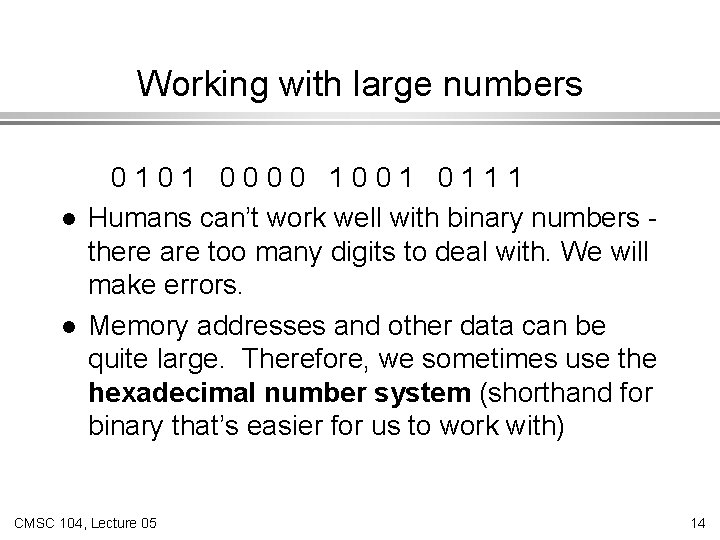Working with large numbers l l 0101 0000 1001 0111 Humans can’t work well with binary numbers there are too many digits to deal with. We will make errors. Memory addresses and other data can be quite large. Therefore, we sometimes use the hexadecimal number system (shorthand for binary that’s easier for us to work with) CMSC 104, Lecture 05 14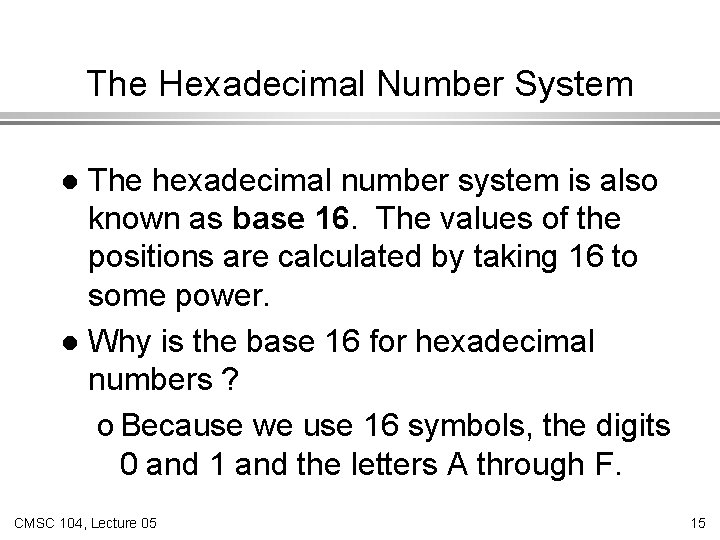The Hexadecimal Number System The hexadecimal number system is also known as base 16. The values of the positions are calculated by taking 16 to some power. l Why is the base 16 for hexadecimal numbers ? o Because we use 16 symbols, the digits 0 and 1 and the letters A through F. l CMSC 104, Lecture 05 15The Hexadecimal Number System (con’t) Binary 0 1 10 11 100 101 110 111 1000 1001 Decimal Hexadecimal 0 0 1 2 3 4 5 6 7 8 9 CMSC 104, Lecture 05 Binary Decimal Hexadecimal 1010 10 A 1011 1100 1101 1110 1111 11 12 13 14 15 B C D E F 16The Hexadecimal Number System (con’t) l Example of a hexadecimal number and the values of the positions: 3 C 8 B 0 5 1 166 165 164 163 162 161 160 CMSC 104, Lecture 05 17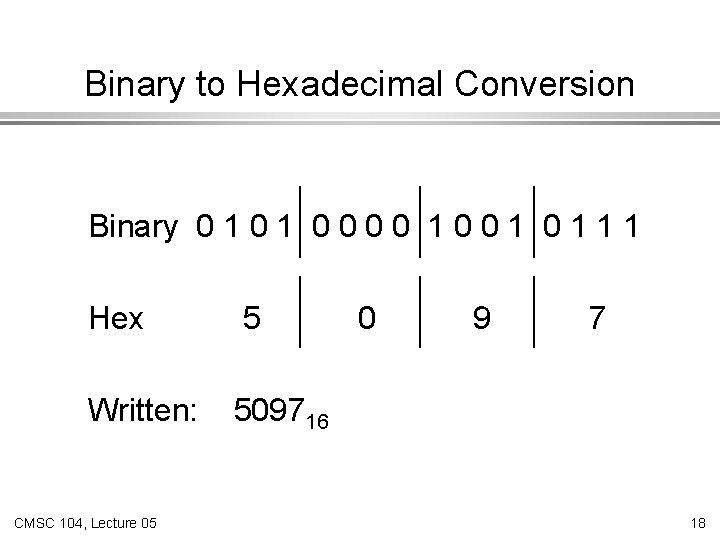Binary to Hexadecimal Conversion Binary 0 1 0 0 1 1 1 Hex 5 Written: 509716 CMSC 104, Lecture 05 0 9 7 18What is Hexadecimal really ? Binary 0 1 0 0 1 1 1 Hex 5 0 9 7 A number expressed in base 16. It’s easy to convert binary to hex and hex to binary because 16 is 24. CMSC 104, Lecture 05 19Another Binary to Hex Conversion Binary 0 1 1 1 0 0 1 1 1 Hex 7 C 3 F 7 C 3 F 16 CMSC 104, Lecture 05 20Summary of Number Systems l l l Binary is base 2, because we use two digits, 0 and 1 Decimal is base 10, because we use ten digits, 0 through 9. Hexadecimal is base 16. How many digits do we need to express numbers in hex ? 16 (0 through F: 0 1 2 3 4 5 6 7 8 9 A B C D E F) CMSC 104, Lecture 05 21Example of Equivalent Numbers Binary: 1 0 0 0 0 1 1 12 Decimal: 2064710 Hexadecimal: 50 A 716 Notice how the number of digits gets smaller as the base increases. CMSC 104, Lecture 05 22